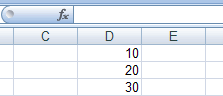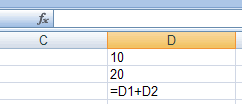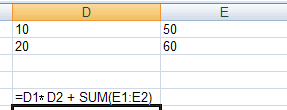## 4. Formulae

 DEFINITION: A formula performs calculations using numbers, addresses of cells and mathematical operators

Formulas are the bread and butter of spreadsheets. Without formulas, a spreadsheet would only contain a static never-changing set of numbers. Not exactly useful.

A formula takes a set of values, usually from other cells, and carries out some maths on them. The result is displayed in the same cell containing the formula.

Normally formulas are not visible when just viewing a spreadsheetThe image on the left is what you would normally see in a spreadsheet. But if you set the spreadsheet software to make formulas visible, then you see the image on the right. Just how you make formulas visible depends on the package, so check with the help menu.

A spreadsheet formula must start with an equals sign (=). It is the trigger to tell the spreadsheet that a calculation is needed.

Formulas can also contain functions which are explained in a later page. Like this.Do you know how to write basic formulae?

Make sure that you know how to write a formula to add, subtract, multiply and divide

Challenge see if you can find out one extra fact on this topic that we haven't already told you